Pure and Applied Mathematics Journal
Volume 4, Issue 5, October 2015, Pages: 216-218

The Simulation of a Queueing System Consist of Two Parallel Heterogeneous Channels with no Waiting Line

Vedat Sağlam1, *, Murat Sağır1, Erdinç Yücesoy1, Müjgan Zobu2

1Department of Statistics, Ondokuz Mayıs University, Samsun, Turkey

2Department of Statistics, Amasya University, Amasya, Turkey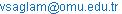(V. Sağlam)

Vedat Sağlam, Murat Sağır, Erdinç Yücesoy, Müjgan Zobu. The Simulation of a Queueing System Consist of Two Parallel Heterogeneous Channels with no Waiting Line.Pure and Applied Mathematics Journal.Vol.4, No. 5, 2015, pp. 216-218. doi: 10.11648/j.pamj.20150405.12

Abstract: A queueing system with two parallel heterogenous channels without waiting is considered. In this queueing system customer arrivals are Poisson distributed withrate. Each customer has exponentially distributed service time withparameter at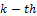channel. When a customer arrives this system if both the service channels are available, the customer has service with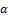or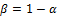probabilities at first and second service channels respectively. If one of the service channels is available, the customer has service at this service channel or leaves the system without being served if both of the service channels are busy. We have obtained mean waiting time and mean number of customers of the system and a simulation of this system is performed.

Keywords: Heterogeneous Queueing Systems, Loss Probability, Optimization, Simulation, Markov Chain, Kolmogorov Equation, Transition Rates

Contents

1. Introduction

Stochastic queueing models and their applications have important roles in fields such as transportation, computer networks, communication, production lines and etc. There are a lot of studies regarding queueing systems with homogeneous Poisson arrivals. In fact in real life practical applications the arrivals to queueing systems are non-homogeneous. The mean customer number and the distribution of waiting time in a tandem queueing system with Poisson arrivals are obtained in . The response time of a tandem Markovian queue with blocking is studied in . A heterogeneous stochastic queueing system with two channel in which no waiting is allowed is analyzed by . Performance measures of a tandem queueing system with two stages are obtained and these performance measures are given in . A queueing model with non-homogeneous bulk arrivals under parallel and series configuration is developed and analyzed in . A number of different models for Markov-modulated queueing systems surveyed. Then a model in which the workload process and the secondary process together constitute a Markov compound Poisson process is analyzed by . In  a tandem queueing system that can be formulated as a continuous-time Markov chain is considered and this system is investigated how to maximize the throughput when the queue is considered and several monotonicity properties of optimal policies for such a system are proved in . The algorithms for the Multi-Server Queue is had in . A queueing system with two parallel heterogeneous channels without waiting is considered in , in this system the arrivals are Poisson distributed withrate. Each customer has exponentially distributed service time with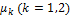parameter at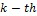channel. When a customer arrives this system if both the service channels are available, the customer has service with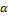or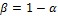probabilities at first and second service channels respectively. If one of the service channels is available, the customer has service at this service channel or leaves the system without being served if both of the service channels are busy so loss occurs. This loss probability optimized in . In this paper we obtain mean waiting time and mean number of customers of the system studied in . In addition, the mean waiting time of this system is optimized under customized conditions. Finally a 100.000 step simulation is done for this system and it is seen that the simulation results tend to exact results.

2. Definition of the Queueing System

The customers arrive this queueing system according to Poisson distribution withparameter. Inservice, each customer has exponentially distributed service time withservice parameter. When a customer arrives this system if both the services are available the customer has service withandprobabilities at first and second service channels respectively. If one of the service channel is available the customer has service at this service channel or leaves the system without being served if both of the service channels are busy so loss occurs. The system is shown in Figure 1 below.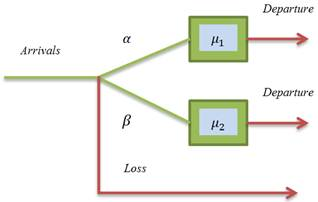Figure 1. A heterogeneous queueing system with parallel channels without waiting line.

3. Kolmogorov Equations

Transient Solutions in Markovian queueing systems are obtained in . Two dimensional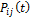Markov chain defines the probability that there arecustomers at first service channel and there arecustomers at second service channel at a given time. Where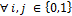. The Kolmogorov differential equations of these transient probabilities are obtained as below: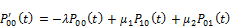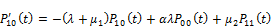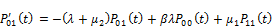(1)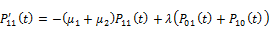where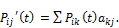(2)

The transition rates matrices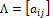is: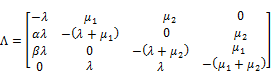(3)

in which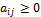and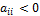and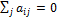Transient probabilities of the system above are found as: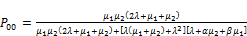(4)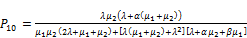(5)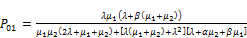(6)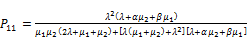(7)

Erlang B formula is given in [10, 11, 14]. The loss probability in queuing systems with heterogeneous servers is shown by [15, 16]. Where,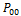is the probability that the system is empty and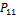is the probability that first and second service channels are busy also this is the loss probability. The optimization of loss probability is as follows:

When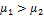and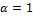then loss probability is minimum .

When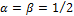under condition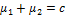the loss probability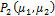takes its minimum value for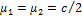.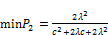(8)

as a result homogeneous system is better than heterogeneous system forcriteria .

4. Performance Measures of System

Performance measure of since there is no waiting in this queueing system, the mean customer number and the mean waiting time in the system is found.

Mean customer number in the system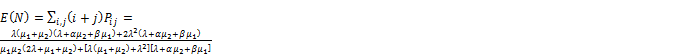(9)

Mean waiting number in the system and its optimization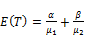(10)

Similarly, optimizations for mean waiting time in the system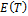can be done. The optimizations done for loss probability above are also valid for.

Whenand, the loss probability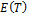is minimum.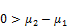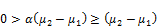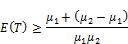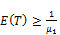(11)

For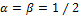and under condition, the loss probabilityhas its minimum value for.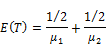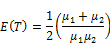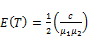(12)

To makeminimum,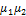product must be maximum. Under condition,product is maximum for.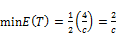(13)

5. Simulation

Table 1. Mean waiting time in the system and loss Probability for.

 Iteration Numbers Simulation Value Exact Value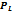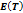500 0.0620 0.6579 0,07770 0,68493 1000 0.0540 0.6790 0,07770 0,68493 5000 0.0606 0.7034 0,07770 0,68493 10000 0.0590 0.6885 0,07770 0,68493

Table 2. Mean waiting time in the system and loss Probability for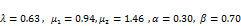.

 Iteration Numbers Simulation Value Exact Value500 0.0820 0.8219 0,08236 0,79860 1000 0.0810 0.8012 0,08236 0,79860 5000 0.0836 0.7942 0,08236 0,79860 10000 0.0718 0.7955 0,08236 0,79860

Table 3. Mean waiting time in the system and loss Probability for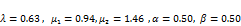.

 Iteration Numbers Simulation Value Exact Value500 0.0860 0.8571 0,08529 0,87438 1000 0.0850 0.8564 0,08529 0,87438 5000 0.0824 0.8504 0,08529 0,87438 10000 0.0858 0.8422 0,08529 0,87438

Table 4. Mean waiting time in the system and loss probability for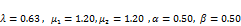.

 Iteration Numbers Simulation Value Exact Value500 0.0820 0.8289 0,08288 0,83333 1000 0.0820 0.8217 0,08288 0,83333 5000 0.0828 0.8383 0,08288 0,83333 10000 0.0824 0.8304 0,08288 0,83333

6. Conclusion

Performance measures, the mean waiting time and the mean number of customers are obtained in this paper. In addition the optimization of mean waiting time is done under conditions given as,, the loss probability and forand under condition.

Loss probability and mean waiting time in this system is obtained by 500, 1000, 5000 and 10000 iteration steps and these results are given in Table1, Table2, Table3 and Table4 respectively. Then it is seen that these simulation results tend to exact values. The number of service channels in the system can be adjusted or a waiting line can be added to this system for further studies.

References

1. Jackson, R. R. P., "Queueing systems with Phase-Type Service", Operat. Res. Quart, 5, 109-120, 1954.
2. J. R. Artalejo and G. Choudhury, "Steady State Analysis of an M/G/1 Queue with Repeated Attempts and Two-Phase Service", Quality Technology&Quantative Management, Vol.1, No.2, pp. 189-199, 2004.
3. M. Zobu, V. Sağlam, M. Sağır, E. Yücesoy and T. Zaman, "The Simulation and Minimization of Loss Probability in the Tandem Queueing with two Heterogeneous Channel", Mathematical problems in Engineering, Vol. 2013, Article ID 529010, 4 pages, 2013.
4. V. Sağlam and M. Zobu, "A two-stage Queueing Model with No waiting Line between Channels", Mathematical Problems in Engineering, Vol. 2013, Article ID 679369, 5 pages, 2013.
5. A. V. S. Suhasini, K. Srinivasa Rao, P. R. S. Reddy, "On parallel and series non homogeneous bulk arrival queueing model", OPSEARCH (Oct-Dec 2013), 50(4):521-547, 2013.
6. N. U. Prabhu and Yixin ZHU, "Markov-modulated Queueing Systems", Queueing System, 5 (1989) 215-246, 1989.
7. Frederick S. Hillier, Kut C. So, "On the optimal design of tandem queueing systems with finite buffers", Queueing Systems 21 (1995) 245-266, 1995.
8. V. V. Rykov, "Monotone Control of Queueing Systems with Heterogeneous Servers", Queueing Systems, 37, 391-403, 2001.
9. V. Sağlam and H. Torun, " On Optimization of Stochastic Service System with Two Heterogeneous Channels", International Journal of Applied Mathematics, 17, 1, 8 / 2005
10. Gross, D., Harris, C. M., Thompson, M. J., Shortle, F. J., Fundementals of Queueing Theory, 4th ed., John Wiley & Sons, New York, 2008.
11. Stewart, W. J., Probability, Markov Chains, Queues and Simulation, Princeton University Press, United Kingdom, 2009.
12. V. Ramaswami, "Algorithms for the Multi-Server Queue", Commun. Statist.-Stochastic Models, 1(3), 393-417, 1985.
13. W. K. Grassmann, "Transient Solutions in Markovian queueing systems", Computers & Operations Research, Volume 4, Issue 1, pages 47-53, 1977.
14. E. Brockmeyer, H. L. Halstrom and A. Jensen, "The life and works of A. K. Erlann." Danish Acad. Techn. Sci, No. 2,  Koben havn (Denmark), 1948.
15. F. Alpaslan, "On the minimization probability of loss in queue two heterogeneous channels,"Pure and Applied Mathematika Sciences, vol. 43, no. 1-2, pp. 21–25, 1996.
16. V. Saglam and A. Shahbazov, "Minimizing loss probability in queuing systems with heterogeneous servers," Iranian Journal of Science and Technology, vol. 31, no. 2, pp. 199–206, 2007.

 Contents 1. 2. 3. 4. 5. 6.
Article ToolsAbstractPDF(299K)